# Proportional Hazards

We will explore the relationship between survival and explanatory variables by modeling. In this class, we consider a broad class of regression models: Proportional Hazards (PH) models. $\log\lambda(t;{\bf Z})=\log\lambda_0(t)+{\boldsymbol\beta}{\bf Z}$ Suppose $$Z = 1$$ for treated subjects and $$Z = 0$$ for untreated subjects. Then this model says that the hazard is increased by a factor of $$e^{\beta}$$ for treated subjects versus untreated subjects ($$e^{\beta}$$ might be < 1).

This group of PH models divides further into:

• Parameteric PH models: Assume parametric form for $$\lambda_0(t)$$, e.g., Weibull distribution.
• Semi-parametric PH models: No assumptions on $$\lambda_0(t)$$: Cox PH model.
library(survival)
library(KMsurv)


# Cox Proportional Hazards model

$\lambda(t;{\bf Z})=\lambda_0(t)\exp\{{\boldsymbol\beta}{\bf Z}\}$

Why do we call it proportional hazards? Think of the first example, where $$Z = 1$$ for treated and $$Z = 0$$ for control. Then if we think of $$\lambda_1(t)$$ as the hazard rate for the treated group, and $$\lambda_0(t)$$ as the hazard for control, then we can write: $\lambda_1(t) = \lambda(t;Z=1)=\lambda_0(t)\exp\{\beta Z\} = \lambda_0(t)\exp\{\beta\}$ This implies that the ratio of the two hazards is a constant, $$\phi$$, which does NOT depend on time, t. In other words, the hazards of the two groups remain proportional over time. $\phi = \frac{\lambda_1(t)}{\lambda_0(t)} = e^{\beta}$ $$\phi$$ is referred to as the hazard ratio.

## Baseline Hazard Function

In the example of comparing two treatment groups, $$\lambda_0(t)$$ is the hazard rate for the control group.

In general, $$\lambda_0(t)$$ is called the baseline hazard function, and reflects the underlying hazard for subjects with all covariates $$Z_1,\ldots,Z_p$$ equal to 0 (i.e., the 'reference group').

The general form is: $\lambda(t;{\bf Z})=\lambda_0(t)\exp\{\beta_1Z_1+\ldots+\beta_pZ_p\}$ So when we substitute all of the $$Z_j$$ equal to 0, we get $$\lambda(t;{\bf Z})=\lambda_0(t)$$.

In the general case, we think of the i-th individual having a set of covariates $${\bf Z}_i = (Z_{1i}, Z_{2i},\ldots, Z_{pi})$$, and we model their hazard rate as some multiple of the baseline hazard rate: $\lambda(t;{\bf Z}_i)=\lambda_0(t)\exp\{\beta_1Z_{1i}+\ldots+\beta_pZ_{pi}\}$ This means we can write the log of the hazard ratio for the i-th individual to the reference group as: $\log\frac{\lambda(t;{\bf Z}_i)}{\lambda_0(t)}=\beta_1Z_{1i}+\ldots+\beta_pZ_{pi}$ The Cox Proportional Hazards model is a linear model for the log of the hazard ratio.

One of the biggest advantages of the framework of the Cox PH model is that we can estimate the parameters $${\boldsymbol\beta}$$ which reflect the effects of treatment and other covariates without having to make any assumptions about the form of $$\lambda_0(t)$$.

In other words, we don???t have to assume that $$\lambda_0(t)$$ follows an exponential model, or a Weibull model, or any other particular parametric model. That???s what makes the model semi-parametric.

Questions:

1. Why don't we just model the hazard ratio, $$\phi = \frac{\lambda_i(t)}{\lambda_0(t)}$$, directly as a linear function of the covariates $${\bf Z}$$?
2. Why doesn't the model have an intercept?

Parameters of interest, $${\boldsymbol\beta}$$, are estimated via partial likelihood. $$\lambda_0(\cdot)$$ is treated as a nuisance parameter.

leuk = read.table("http://www.karlin.mff.cuni.cz/~pesta/prednasky/NMFM404/Data/leuk.dat", sep=" ", head=T, skip=7) # read data

##   weeks remiss trtmt
## 1     6      0     1
## 2     6      1     1
## 3     6      1     1
## 4     6      1     1
## 5     7      1     1
## 6     9      0     1

# Cox PH Model
leuk.ph = coxph(Surv(weeks,remiss) ~ trtmt, data=leuk)
# Note: default = Efron method for handling ties
summary(leuk.ph)

## Call:
## coxph(formula = Surv(weeks, remiss) ~ trtmt, data = leuk)
##
##   n= 42, number of events= 30
##
##          coef exp(coef) se(coef)      z Pr(>|z|)
## trtmt -1.5721    0.2076   0.4124 -3.812 0.000138 ***
## ---
## Signif. codes:  0 '***' 0.001 '**' 0.01 '*' 0.05 '.' 0.1 ' ' 1
##
##       exp(coef) exp(-coef) lower .95 upper .95
## trtmt    0.2076      4.817   0.09251    0.4659
##
## Concordance= 0.69  (se = 0.053 )
## Rsquare= 0.322   (max possible= 0.988 )
## Likelihood ratio test= 16.35  on 1 df,   p=5.261e-05
## Wald test            = 14.53  on 1 df,   p=0.0001378
## Score (logrank) test = 17.25  on 1 df,   p=3.283e-05

# Breslow handling of ties
leuk.phb = coxph(Surv(weeks, remiss) ~ trtmt, data=leuk, method="breslow")
summary(leuk.phb)

## Call:
## coxph(formula = Surv(weeks, remiss) ~ trtmt, data = leuk, method = "breslow")
##
##   n= 42, number of events= 30
##
##          coef exp(coef) se(coef)      z Pr(>|z|)
## trtmt -1.5092    0.2211   0.4096 -3.685 0.000229 ***
## ---
## Signif. codes:  0 '***' 0.001 '**' 0.01 '*' 0.05 '.' 0.1 ' ' 1
##
##       exp(coef) exp(-coef) lower .95 upper .95
## trtmt    0.2211      4.523   0.09907    0.4934
##
## Concordance= 0.69  (se = 0.053 )
## Rsquare= 0.304   (max possible= 0.989 )
## Likelihood ratio test= 15.21  on 1 df,   p=9.615e-05
## Wald test            = 13.58  on 1 df,   p=0.0002288
## Score (logrank) test = 15.93  on 1 df,   p=6.571e-05

# Exact handling of ties
leuk.phe = coxph(Surv(weeks, remiss) ~ trtmt, data=leuk, method="exact")
summary(leuk.phe)

## Call:
## coxph(formula = Surv(weeks, remiss) ~ trtmt, data = leuk, method = "exact")
##
##   n= 42, number of events= 30
##
##          coef exp(coef) se(coef)      z Pr(>|z|)
## trtmt -1.6282    0.1963   0.4331 -3.759  0.00017 ***
## ---
## Signif. codes:  0 '***' 0.001 '**' 0.01 '*' 0.05 '.' 0.1 ' ' 1
##
##       exp(coef) exp(-coef) lower .95 upper .95
## trtmt    0.1963      5.095   0.08398    0.4587
##
## Rsquare= 0.321   (max possible= 0.98 )
## Likelihood ratio test= 16.25  on 1 df,   p=5.544e-05
## Wald test            = 14.13  on 1 df,   p=0.0001704
## Score (logrank) test = 16.79  on 1 df,   p=4.169e-05


### Adjustments for ties

The proportional hazards model assumes a continuous hazard ??? ties are not possible. There are four proposed modifications to the likelihood to adjust for ties.

Bottom Line: Implications of Ties (See Allison (1995), p.127-137)

• When there are no ties, all options give exactly the same results.
• When there are only a few ties, it won???t make much difference which method is used. However, since the exact methods won't take much extra computing time, you might as well use one of them.
• When there are many ties (relative to the number at risk), the Breslow option (default) performs poorly (Farewell & Prentice, 1980; Hsieh, 1995). Both of the approximate methods, Breslow and Efron, yield coefficients that are attenuated (biased toward 0).
• The choice of which exact method to use should be based on substantive grounds - are the tied event times truly tied? ... or are they the result of imprecise measurement?
• Computing time of exact methods is much longer than that of the approximate methods. However, in most cases it will still be less than 30 seconds even for the exact methods.
• Best approximate method - the Efron approximation nearly always works better than the Breslow method, with no increase in computing time, so use this option if exact methods are too computer-intensive.

# Confidence intervals and hypothesis tests

Many software packages provide estimates of $${\boldsymbol\beta}$$, but the hazard ratio $$HR=\exp\{{\boldsymbol\beta}\}$$ is usually the parameter of interest.

For each covariate of interest, the null hypothesis is $H_0:\,HR_j=1\,\Leftrightarrow\,\beta_j=0$ Wald test is used. For nested models, a likelihood ratio test is constructed.

## MAC prevention clinical trial data

ACTG 196 was a randomized clinical trial to study the effects of combination regimens on prevention of MAC (mycobacterium avium complex), one of the most common opportunistic infections in AIDS patients.

The treatment regimens were:

• clarithromycin (new)
• rifabutin (standard)
• clarithromycin plus rifabutin
mac = read.csv("http://www.karlin.mff.cuni.cz/~pesta/prednasky/NMFM404/Data/mac.csv", head=F, col.names =
c("patid", "age", "agecat", "sex", "karnof", "ivdrug",
"antiret", "cd4", "cd4cat", "ctg", "dthstat", "dthtime",
"macstat", "mactime", "swdrstat", "swdrtime", "rif",
"clari", "toxstat", "toxtime"))
# mactime = time to mac
# macstat = event indicator
head(mac)     # Check out a few data rows:

##   patid age agecat sex karnof ivdrug antiret cd4 cd4cat ctg dthstat
## 1     1  42      1   0     90      0       1   8      0   1       0
## 2     2  33      0   0     90      0       0  30      1   1       0
## 3     3  39      1   0    100      0       1  80      1   1       1
## 4     4  35      0   0     80      0       0  58      1   1       0
## 5     5  42      1   0     90      0       0  59      1   1       0
## 6     6  45      1   0     90      0       0  18      0   1       1
##   dthtime macstat mactime swdrstat swdrtime rif clari toxstat toxtime
## 1     623       1     560        1      106   1     0       0     623
## 2     651       0     651        1       86   1     0       0     651
## 3     464       0      26        0       26   1     0       0      26
## 4     622       0     622        1      111   0     1       0     501
## 5     643       0     643        1      119   0     1       0     643
## 6     216       0     171        0      171   0     1       1     171

# Treatment arms:
#  rif + clari (N=389)
#  clari (N=397)
#  rif (N=391)
table(mac$clari, mac$rif)

##
##       0   1
##   0 389 391
##   1 397   0

# Fit and plot KM survival curves
fit.km = survfit(Surv(mactime, macstat) ~ clari + rif, data=mac)


plot(fit.km, mark.time=F, lty=1:3, xlab="Days", ylab="MAC-Free Survival")
legend(lty=1:3, x=100, y=.4, legend=c("Rif + Clari", "Rifabutin", "Clarithromycin"), cex=0.8)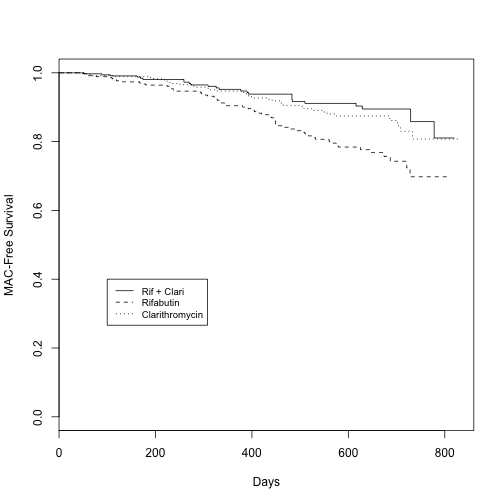Model 1

# Fit Cox PH model
fit.mac1 = coxph(Surv(mactime,macstat) ~ karnof + clari + rif, data=mac)
summary(fit.mac1)

## Call:
## coxph(formula = Surv(mactime, macstat) ~ karnof + clari + rif,
##     data = mac)
##
##   n= 1177, number of events= 121
##
##            coef exp(coef) se(coef)      z Pr(>|z|)
## karnof -0.04485   0.95614  0.01064 -4.217 2.48e-05 ***
## clari   0.27557   1.31728  0.25801  1.068 0.285509
## rif     0.87197   2.39161  0.23694  3.680 0.000233 ***
## ---
## Signif. codes:  0 '***' 0.001 '**' 0.01 '*' 0.05 '.' 0.1 ' ' 1
##
##        exp(coef) exp(-coef) lower .95 upper .95
## karnof    0.9561     1.0459    0.9364    0.9763
## clari     1.3173     0.7591    0.7944    2.1842
## rif       2.3916     0.4181    1.5032    3.8051
##
## Concordance= 0.649  (se = 0.028 )
## Rsquare= 0.027   (max possible= 0.73 )
## Likelihood ratio test= 32.02  on 3 df,   p=5.193e-07
## Wald test            = 32.29  on 3 df,   p=4.548e-07
## Score (logrank) test = 33.16  on 3 df,   p=2.977e-07


Model 2

# Fit Cox PH model adding baseline CD4 variable
fit.mac2 = coxph(Surv(mactime,macstat) ~ karnof + clari + rif + cd4, data=mac)
summary(fit.mac2)

## Call:
## coxph(formula = Surv(mactime, macstat) ~ karnof + clari + rif +
##     cd4, data = mac)
##
##   n= 1177, number of events= 121
##
##             coef exp(coef)  se(coef)      z Pr(>|z|)
## karnof -0.036874  0.963798  0.010665 -3.457 0.000546 ***
## clari   0.252345  1.287041  0.258337  0.977 0.328664
## rif     0.879749  2.410294  0.237092  3.711 0.000207 ***
## cd4    -0.018360  0.981807  0.003684 -4.984 6.23e-07 ***
## ---
## Signif. codes:  0 '***' 0.001 '**' 0.01 '*' 0.05 '.' 0.1 ' ' 1
##
##        exp(coef) exp(-coef) lower .95 upper .95
## karnof    0.9638     1.0376    0.9439    0.9842
## clari     1.2870     0.7770    0.7757    2.1354
## rif       2.4103     0.4149    1.5145    3.8360
## cd4       0.9818     1.0185    0.9747    0.9889
##
## Concordance= 0.716  (se = 0.028 )
## Rsquare= 0.053   (max possible= 0.73 )
## Likelihood ratio test= 63.77  on 4 df,   p=4.682e-13
## Wald test            = 55.59  on 4 df,   p=2.449e-11
## Score (logrank) test = 56.22  on 4 df,   p=1.806e-11


We can also compute the hazard ratio ourselves, by exponentiating the $$\beta$$ coefficients: $HR_{cd4} = \exp\{???0.01835\} = 0.98$ Why is this HR so close to 1, and yet still highly significant? What is the interpretation of this HR?

The likelihood ratio test for the effect of CD4 is twice the difference in minus log-likelihoods between the two models (on 1 df): $??^2{LR} = 2???(63.8???32.0)=31.8$ How does this test statistic compare to the Wald $$\chi^2$$ test?

In the mac study, there were three treatment arms (rif, clari, and the rif+clari combination (for rif=clari=0). The combination therapy is the reference group, so the coefficients of rif and clari are in comparison to the combination group.

How do we compare the Rifa arm against the Clari arm? That is equivalent to testing $H_0:\,\beta_{clari}=\beta_{rifa}$ For that, we need the matrix $$\mathsf{var}\widehat{\boldsymbol\beta}$$.

names(fit.mac2)   # check components of fit object

##   "coefficients"      "var"               "loglik"
##   "score"             "iter"              "linear.predictors"
##   "residuals"         "means"             "concordance"
##  "method"            "n"                 "nevent"
##  "terms"             "assign"            "wald.test"
##  "y"                 "formula"           "call"

beta_hat = fit.mac2$coef Var_beta = fit.mac2$var   # Var(beta_hat)
A = c(0,1,-1,0)   # contrast vector
beta_dif = t(A) %*% beta_hat     # beta_clari - beta_rif
var_dif = t(A) %*% Var_beta %*% A   # Var(beta_clari-beta_rif)
se_dif = sqrt(var_dif)        # SE(beta_clari-beta_rif)
t_dif = beta_dif/se_dif
t_dif     # Wald test t-statistic, df=n-p

##           [,1]
## [1,] -2.959871

2* pt( -abs(t_dif), df=1177-4) # p-value for difference in arms

##             [,1]
## [1,] 0.003139506


Testing Model 2 for overall treatment effect Two ways:

1. Wald test. $$\beta_{rif} = 0\,\&\,\beta_{clari} = 0$$. Null distribution is Chi-square with df=2 (why?).
A = matrix(0, ncol=4, nrow=2)
A[1,2] = A[2,3] = 1
beta_trt = A %% beta_hat
var_trt = A %% Var_beta %% t(A)
(Wald_trt = t(beta_trt) %% solve(var_trt) %*% beta_trt)

##          [,1]
## [1,] 17.00252

1-pchisq(Wald_trt, df=2)  # p-value for Wald test for treatment

##              [,1]
## [1,] 0.0002032125

2. Likelihood ratio test. Fit model with and without rif, clari and compare log-likelihood, against Chi-square with df=2.
fit.mac3 = update(fit.mac2, .~. -rif -clari)
anova(fit.mac3, fit.mac2, test="Chisq")

## Analysis of Deviance Table
##  Cox model: response is  Surv(mactime, macstat)
##  Model 1: ~ karnof + cd4
##  Model 2: ~ karnof + clari + rif + cd4
##    loglik  Chisq Df P(>|Chi|)
## 1 -747.07
## 2 -738.62 16.914  2 0.0002125 ***
## ---
## Signif. codes:  0 '**' 0.001 '' 0.01 '' 0.05 '.' 0.1 ' ' 1


# Predicted survival

For the i-th patient with covariates $${\bf Z}_i$$, we have: $S_i(t)=[S_0(t)]^{\exp\{{\boldsymbol\beta}{\bf Z}_i\}}$ Say we are interested in the survival pattern for single males in the nursing home study. Based on the previous formula, if we had an estimate for the survival function in the reference group, i.e., $$\widehat{S}_0(t)$$, we could get estimates of the survival function for any set of covariates $${\bf Z}_i$$.

How can we estimate the survival function, $$S_0(t)$$? We could use the KM estimator, but there are a few disadvantages of that approach:

• It would only use the survival times for observations contained in the reference group, and not all the rest of the survival times.
• It would tend to be somewhat choppy, since it would reflect the smaller sample size of the reference group.
• It???s possible that there are no subjects in the dataset who are in the 'reference' group (ex. say covariates are age and sex; there is no one of age=0 in our dataset).

Instead, we will use a baseline hazard estimator which takes advantage of the proportional hazards assumption to get a smoother estimate. $\widehat{S}_i(t)=[\widehat{S}_0(t)]^{\exp\{\widehat{\boldsymbol\beta}{\bf Z}_i\}}$ Using the above formula, we substitute $$\widehat{\boldsymbol\beta}$$ based on fitting the Cox PH model, and calculate $$\widehat{S}_0(t)$$ by one of the following approaches:

• Breslow estimator (R) ... extending the concept of the Nelson-Aalen estimator to the proportional hazards model
• Kalbfleisch/Prentice estimator (R, SAS) ... analogous to the Kaplan-Meier estimator

Consider the Nursing Home study. We fit the model $\lambda_(t) = \lambda_0(t) \exp\{\beta_1[\mbox{married}] + \beta_2[\mbox{health status}]\}$ Let's predict the survival for single individuals with health status = 5 (worst), and for those with health status = 4 (second worst).

nurshome = read.table("http://www.karlin.mff.cuni.cz/~pesta/prednasky/NMFM404/Data/NursingHome.dat", head=F, skip=14, col.names = c("los", "age", "rx", "gender", "married", "health", "censor"))
nurshome$discharged = 1 - nurshome$censor # event indicator

##    los age rx gender married health censor discharged
## 1   37  86  1      0       0      2      0          1
## 2   61  77  1      0       0      4      0          1
## 3 1084  75  1      0       0      3      1          0
## 4 1092  77  1      0       1      2      1          0
## 5   23  86  1      0       0      4      0          1
## 6 1091  71  1      1       0      3      1          0

table(nurshome$health)  ## ## 2 3 4 5 ## 343 576 513 169  # Fit Cox PH model fit.nurs = coxph(Surv(los,discharged) ~ married + health, data=nurshome) # Predict survival for single patients with health = 5 newdat = data.frame(married=0, health=5) newdat # data frame with same predictors as fit.nurs  ## married health ## 1 0 5  ps5 = survfit(fit.nurs, newdata=newdat) # predicted survival # Compare with Kaplan-Meier nursh5 = nurshome[nurshome$health==5 & nurshome$married==0,] fit.km5 = survfit(Surv(los, discharged) ~ 1, data = nursh5) # Predict survival for single patients, health =4 newdat[1,] = c(0,4) ps4 = survfit(fit.nurs, newdata=newdat) # Compare with Kaplan-Meier nursh4 = nurshome[nurshome$health==4 & nurshome$married==0,] fit.km4 = survfit(Surv(los, discharged) ~ 1, data = nursh4)  plot(ps5, xlab= "Length of stay in nursing home (days)", ylab = "Proportion not discharged", col=2, conf.int=F) lines(fit.km5, mark.time=F, col=2) # add line to existing plot lines(ps4, xlab= "Length of stay in nursing home (days)", ylab = "Proportion not discharged", col=4, conf.int=F) lines(fit.km4, mark.time=F, col=4) # add line to existing plot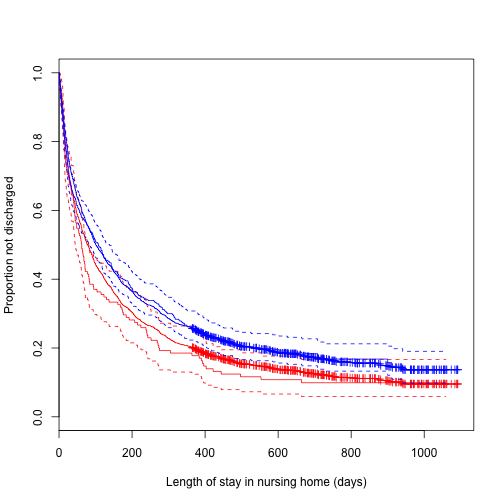Continuing the Nursing Home example, let's get and plot the baseline survival $$S_0(t)$$ and cumulative hazard $$\Lambda_0(t)$$. They correspond to married=0, health=0. newdat[1,] = c(0,0) ps0 = survfit(fit.nurs, newdata=newdat) # S_0 = ps0$surv
bh = basehaz(fit.nurs, centered=F)
Lambda0 = bh$hazard # it???s *cummulative* hazard! # same as Lambda0 = -log(ps0$surv)


plot(bh$time, Lambda0, type="l", xlab="Length of stay in nursing home (days)", ylab="Cummulative hazard", col=4)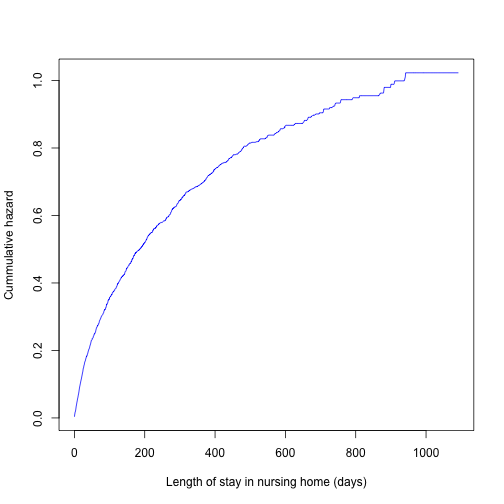# Model selection Collett (Section 3.6) has an excellent discussion of various approaches for model selection. In practice, model selection proceeds through a combination of • knowledge of the science • trial and error, common sense • automatic variable selection procedures • forward selection • backward selection • stepwise selection Many advocate the approach of first doing a univariate analysis to 'screen' out potentially significant variables for consideration in the multivariate model (see Collett). Moreover, typically univariate analysis is a part of a larger analysis, in order to identify the importance of each predictor taken in itself. ## Atlantic halibut survival times data One conservation measure suggested for trawl fishing is a minimum size limit for halibut (32 inches). However, this size limit would only be effective if captured fish below the limit survived until the time of their release. An experiment was conducted to evaluate the survival rates of halibut caught by trawls or longlines, and to assess other factors which might contribute to survival (duration of trawling, maximum depth fished, size of fish, and handling time). halibut = read.csv("http://www.karlin.mff.cuni.cz/~pesta/prednasky/NMFM404/Data/halibut.csv", head=T) dim(halibut)  ##  294 8  head(halibut)  ## No Time Death Towd Deldepth Length Handtime Logcat ## 1 1 209 1 30 13 41 8 6.992 ## 2 2 209 1 30 13 44 8 6.992 ## 3 3 209 1 30 13 47 10 6.992 ## 4 4 209 1 30 13 34 10 6.992 ## 5 5 38 1 30 13 40 11 6.992 ## 6 6 209 1 30 13 42 11 6.992  # Time = Survival time, in minutes # Death = Event indicator # Length = length of fish (cm) # Handtime = handling time (min) # Logcat = log total catch  ### Univariate analysis # Towing duration: table(halibut$Towd)

##
##  30 100
## 107 187

kmtow = survfit(Surv(Time, Death) ~ Towd, data=halibut)
# Length of fish:
summary(halibut$Length)  ## Min. 1st Qu. Median Mean 3rd Qu. Max. ## 29.00 38.00 43.00 42.51 46.75 57.00  halibut$Lencat = (halibut$Length > 42) + 0 table(halibut$Lencat)

##
##   0   1
## 134 160

kmlen = survfit(Surv(Time, Death) ~ Lencat, data=halibut)
# Depth range of towing:
table(halibut$Deldepth)  ## ## 1 2 3 4 5 6 8 10 13 15 23 49 58 ## 6 17 8 6 45 46 40 68 8 12 32 3 3  summary(halibut$Deldepth)

##    Min. 1st Qu.  Median    Mean 3rd Qu.    Max.
##   1.000   5.000   8.000   9.966  10.000  58.000

halibut$Depthcat = cut(halibut$Deldepth, breaks=c(0,5,9,11,60))
kmdep = survfit(Surv(Time, Death) ~ Depthcat, data=halibut)
# Handling time:
summary(halibut$Handtime)  ## Min. 1st Qu. Median Mean 3rd Qu. Max. ## 1.00 6.00 10.00 12.51 17.00 38.00  halibut$Handcat = cut(halibut$Handtime, breaks = c(0,6,10,17,40)) kmhan = survfit(Surv(Time, Death) ~ Handcat, data=halibut) # Log total catch: summary(halibut$Logcat)

##    Min. 1st Qu.  Median    Mean 3rd Qu.    Max.
##   2.904   4.059   4.203   4.886   5.323   8.690

halibut$Catchcat = cut(halibut$Logcat, breaks=c(0,4.06,4.89,5.33,9))
kmlgc = survfit(Surv(Time, Death) ~ Catchcat, data = halibut)


par(mfrow=c(3,2))
plot(kmtow, lty=1:2, col=2:3, xlab="Minutes", ylab="Survival", main="Towing Duration")
plot(kmlen, lty=1:2, col=2:3, xlab="Minutes", ylab="Survival", main="Fish Length")
plot(kmdep, lty=1:4, col=1:4, xlab="Minutes", ylab="Survival", main="Depth of Towing")
plot(kmhan, lty=1:4, col=1:4, xlab="Minutes", ylab="Survival", main="Handling Time")
plot(kmlgc, lty=1:4, col=1:4, xlab="Minutes", ylab="Survival", main="Total Catch")
par(mfrow=c(1,1))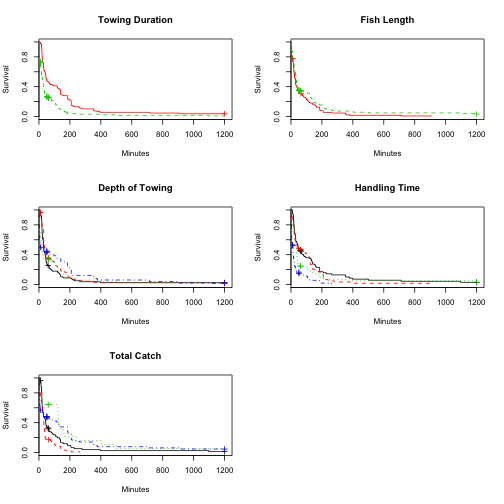## Model selection approach

1. Fit a univariate model for each covariate, and identify the predictors significant at some level $$p_1$$, say 0.20 (Hosmer and Lemeshow recommend $$p_1 = 0.25$$).
2. Fit a multivariate model with all significant univariate predictors, and use backward selection to eliminate nonsignificant variables at some level $$p_2$$, say 0.10.
3. Starting with final step 2. model, consider each of the non-significant variables from step 1. using forward selection, with significance level $$p_3$$, say 0.10.
4. Do final pruning of main-effects model (omit variables that are non-significant, add any that are significant), using stepwise regression with significance level $$p_4$$. At this stage, you may also consider adding interactions between any of the main effects currently in the model, under the hierarchical principle.

Collett recommends using a likelihood ratio test for all variable inclusion/exclusion decisions.

Each step uses a 'greedy' approach:

• Backward step: among several candidates for elimi- nation from the model, the one with the smallest effect on the criterion (AIC, LR), is eliminated None is eliminated if AIC increases (AIC) or LR significant (LR)
• Forward step: among several candidates for addition to the model, the one with the largest effect on the criterion (AIC, LR), is added None is added if AIC increases (AIC) or LR not significant (LR)
library(MASS)
args(stepAIC)

## function (object, scope, scale = 0, direction = c("both", "backward",
##     "forward"), trace = 1, keep = NULL, steps = 1000, use.start = FALSE,
##     k = 2, ...)
## NULL

# Model selection for Halibut data
fit = coxph(Surv(Time, Death) ~ Towd + Length + Deldepth + Handtime + Logcat, data=halibut)
# Backward selection using AIC
fitb = stepAIC(fit, direction="backward", k=2)

## Start:  AIC=2520.15
## Surv(Time, Death) ~ Towd + Length + Deldepth + Handtime + Logcat
##
##            Df    AIC
## - Deldepth  1 2519.7
## <none>        2520.2
## - Towd      1 2531.7
## - Logcat    1 2532.8
## - Length    1 2533.0
## - Handtime  1 2544.9
##
## Step:  AIC=2519.7
## Surv(Time, Death) ~ Towd + Length + Handtime + Logcat
##
##            Df    AIC
## <none>        2519.7
## - Logcat    1 2530.8
## - Length    1 2531.2
## - Towd      1 2532.7
## - Handtime  1 2547.7

summary(fitb)

## Call:
## coxph(formula = Surv(Time, Death) ~ Towd + Length + Handtime +
##     Logcat, data = halibut)
##
##   n= 294, number of events= 273
##
##               coef exp(coef)  se(coef)      z Pr(>|z|)
## Towd      0.007744  1.007774  0.002017  3.839 0.000123 ***
## Length   -0.036960  0.963715  0.010028 -3.686 0.000228 ***
## Handtime  0.054947  1.056485  0.009870  5.567 2.59e-08 ***
## Logcat   -0.183373  0.832458  0.050982 -3.597 0.000322 ***
## ---
## Signif. codes:  0 '***' 0.001 '**' 0.01 '*' 0.05 '.' 0.1 ' ' 1
##
##          exp(coef) exp(-coef) lower .95 upper .95
## Towd        1.0078     0.9923    1.0038    1.0118
## Length      0.9637     1.0377    0.9450    0.9828
## Handtime    1.0565     0.9465    1.0362    1.0771
## Logcat      0.8325     1.2013    0.7533    0.9199
##
## Concordance= 0.683  (se = 0.02 )
## Rsquare= 0.25   (max possible= 1 )
## Likelihood ratio test= 84.5  on 4 df,   p=0
## Wald test            = 90.71  on 4 df,   p=0
## Score (logrank) test = 94.51  on 4 df,   p=0

# Forward selection using AIC
fit0 = coxph(Surv(Time, Death) ~ 1, data=halibut)
# (starting model)
fitf = stepAIC(fit0, scope=formula(fit), direction="forward", k=2)

## Warning in is.na(fit$coefficients): is.na() applied to non-(list or ## vector) of type 'NULL'  ## Start: AIC=2596.2 ## Surv(Time, Death) ~ 1  ## Warning in is.na(fit$coefficients): is.na() applied to non-(list or
## vector) of type 'NULL'

## Warning in is.na(fit$coefficients): is.na() applied to non-(list or ## vector) of type 'NULL'  ## Df AIC ## + Handtime 1 2556.8 ## + Towd 1 2568.0 ## + Length 1 2586.1 ## + Deldepth 1 2594.5 ## <none> 2596.2 ## + Logcat 1 2596.8 ## ## Step: AIC=2556.85 ## Surv(Time, Death) ~ Handtime ## ## Df AIC ## + Logcat 1 2540.3 ## + Towd 1 2543.6 ## + Length 1 2549.9 ## <none> 2556.8 ## + Deldepth 1 2558.8 ## ## Step: AIC=2540.3 ## Surv(Time, Death) ~ Handtime + Logcat ## ## Df AIC ## + Towd 1 2531.2 ## + Length 1 2532.7 ## <none> 2540.3 ## + Deldepth 1 2541.4 ## ## Step: AIC=2531.23 ## Surv(Time, Death) ~ Handtime + Logcat + Towd ## ## Df AIC ## + Length 1 2519.7 ## <none> 2531.2 ## + Deldepth 1 2533.0 ## ## Step: AIC=2519.7 ## Surv(Time, Death) ~ Handtime + Logcat + Towd + Length ## ## Df AIC ## <none> 2519.7 ## + Deldepth 1 2520.2  summary(fitf)  ## Call: ## coxph(formula = Surv(Time, Death) ~ Handtime + Logcat + Towd + ## Length, data = halibut) ## ## n= 294, number of events= 273 ## ## coef exp(coef) se(coef) z Pr(>|z|) ## Handtime 0.054947 1.056485 0.009870 5.567 2.59e-08 *** ## Logcat -0.183373 0.832458 0.050982 -3.597 0.000322 *** ## Towd 0.007744 1.007774 0.002017 3.839 0.000123 *** ## Length -0.036960 0.963715 0.010028 -3.686 0.000228 *** ## --- ## Signif. codes: 0 '***' 0.001 '**' 0.01 '*' 0.05 '.' 0.1 ' ' 1 ## ## exp(coef) exp(-coef) lower .95 upper .95 ## Handtime 1.0565 0.9465 1.0362 1.0771 ## Logcat 0.8325 1.2013 0.7533 0.9199 ## Towd 1.0078 0.9923 1.0038 1.0118 ## Length 0.9637 1.0377 0.9450 0.9828 ## ## Concordance= 0.683 (se = 0.02 ) ## Rsquare= 0.25 (max possible= 1 ) ## Likelihood ratio test= 84.5 on 4 df, p=0 ## Wald test = 90.71 on 4 df, p=0 ## Score (logrank) test = 94.51 on 4 df, p=0  # same final model as backward selection! # Stepwise model selection (backward and forward) fits = stepAIC(fit, direction="both", k=2)  ## Start: AIC=2520.15 ## Surv(Time, Death) ~ Towd + Length + Deldepth + Handtime + Logcat ## ## Df AIC ## - Deldepth 1 2519.7 ## <none> 2520.2 ## - Towd 1 2531.7 ## - Logcat 1 2532.8 ## - Length 1 2533.0 ## - Handtime 1 2544.9 ## ## Step: AIC=2519.7 ## Surv(Time, Death) ~ Towd + Length + Handtime + Logcat ## ## Df AIC ## <none> 2519.7 ## + Deldepth 1 2520.2 ## - Logcat 1 2530.8 ## - Length 1 2531.2 ## - Towd 1 2532.7 ## - Handtime 1 2547.7  summary(fits)  ## Call: ## coxph(formula = Surv(Time, Death) ~ Towd + Length + Handtime + ## Logcat, data = halibut) ## ## n= 294, number of events= 273 ## ## coef exp(coef) se(coef) z Pr(>|z|) ## Towd 0.007744 1.007774 0.002017 3.839 0.000123 *** ## Length -0.036960 0.963715 0.010028 -3.686 0.000228 *** ## Handtime 0.054947 1.056485 0.009870 5.567 2.59e-08 *** ## Logcat -0.183373 0.832458 0.050982 -3.597 0.000322 *** ## --- ## Signif. codes: 0 '***' 0.001 '**' 0.01 '*' 0.05 '.' 0.1 ' ' 1 ## ## exp(coef) exp(-coef) lower .95 upper .95 ## Towd 1.0078 0.9923 1.0038 1.0118 ## Length 0.9637 1.0377 0.9450 0.9828 ## Handtime 1.0565 0.9465 1.0362 1.0771 ## Logcat 0.8325 1.2013 0.7533 0.9199 ## ## Concordance= 0.683 (se = 0.02 ) ## Rsquare= 0.25 (max possible= 1 ) ## Likelihood ratio test= 84.5 on 4 df, p=0 ## Wald test = 90.71 on 4 df, p=0 ## Score (logrank) test = 94.51 on 4 df, p=0  # Stepwise selection with alpha=k=3 fitk3 = stepAIC(fit, direction="both", k=3)  ## Start: AIC=2525.15 ## Surv(Time, Death) ~ Towd + Length + Deldepth + Handtime + Logcat ## ## Df AIC ## - Deldepth 1 2523.7 ## <none> 2525.2 ## - Towd 1 2535.7 ## - Logcat 1 2536.8 ## - Length 1 2537.0 ## - Handtime 1 2548.9 ## ## Step: AIC=2523.7 ## Surv(Time, Death) ~ Towd + Length + Handtime + Logcat ## ## Df AIC ## <none> 2523.7 ## + Deldepth 1 2525.2 ## - Logcat 1 2533.8 ## - Length 1 2534.2 ## - Towd 1 2535.7 ## - Handtime 1 2550.7  summary(fitk3)  ## Call: ## coxph(formula = Surv(Time, Death) ~ Towd + Length + Handtime + ## Logcat, data = halibut) ## ## n= 294, number of events= 273 ## ## coef exp(coef) se(coef) z Pr(>|z|) ## Towd 0.007744 1.007774 0.002017 3.839 0.000123 *** ## Length -0.036960 0.963715 0.010028 -3.686 0.000228 *** ## Handtime 0.054947 1.056485 0.009870 5.567 2.59e-08 *** ## Logcat -0.183373 0.832458 0.050982 -3.597 0.000322 *** ## --- ## Signif. codes: 0 '***' 0.001 '**' 0.01 '*' 0.05 '.' 0.1 ' ' 1 ## ## exp(coef) exp(-coef) lower .95 upper .95 ## Towd 1.0078 0.9923 1.0038 1.0118 ## Length 0.9637 1.0377 0.9450 0.9828 ## Handtime 1.0565 0.9465 1.0362 1.0771 ## Logcat 0.8325 1.2013 0.7533 0.9199 ## ## Concordance= 0.683 (se = 0.02 ) ## Rsquare= 0.25 (max possible= 1 ) ## Likelihood ratio test= 84.5 on 4 df, p=0 ## Wald test = 90.71 on 4 df, p=0 ## Score (logrank) test = 94.51 on 4 df, p=0  Notes: • When the halibut data was analyzed with the forward, backward and stepwise options, the same final model was reached. However, this will not always (in fact, rarely) be the case. • Variables can be forced into the model using the include option in SAS. Any variables that you want to force in- clusion of must be listed first in your model statement. In stepAIC, these are included in the scope part. • SAS uses the score test to decide what variables to add and the Wald test for what variables to remove. • When might we want to force certain variables into the model? 1. to examine interactions 2. to keep main effects in the model 3. to include scientifically important variables, e.g. treatment, gender, race in a medical study, or other covariates which are known from previous studies or from theory to be important. • Model selection is an imperfect art! • No method is universally agreed upon • Choosing between two models using LR or AIC has an information theory grounding • Forward/backward/stepwise selection have no theo- retical bases • Leads to potential biases, nominal p-values smaller than true p-values • The model selection step is usually not taken into account, and inference is done as if the chosen model were 'true' # Model diagnostics - Assessing overall model fit How do we know if the model fits well? • Always look at univariate plots (Kaplan-Meiers). Construct a Kaplan-Meier survival plot for each of the important predictors. • Check proportional hazards assumption. • Check residuals. 1. generalized (Cox-Snell) 2. martingale 3. deviance 4. Schoenfeld 5. weighted Schoenfeld ## Generalized residuals # Analysis of halibut data, continued # 1. Compute cummulative hazard Lambda_0: Lambda0 = basehaz(fitb, centered=F) Lambda0[1:3,]  ## hazard time ## 1 0.009323417 1.1 ## 2 0.018739005 1.2 ## 3 0.057162782 1.3  # 2. Compute Lambda_0(X_i) for all i: predLambda0 = function(Lambda0, t) { u = length(Lambda0$time[Lambda0$time0, Lambda0$hazard[u], 0) )
}
n = length(halibut$Time) L0 = rep(NA, n) # for storing Lambda_0(X_i) for(i in 1:n) L0[i] = predLambda0(Lambda0, halibut$Time[i])
# 3. Compute exp(beta Z_i)
ebetaZ =  exp(as.matrix(halibut[,c(4,6,7,8)]) %*% fitb$coef) # 4. Compute e_i for all i: e = L0 * ebetaZ # 5. Run KM for (e, delta) resfit = survfit(Surv(e,halibut$Death) ~ 1)


# 6.1 Plot log survival function against time
plot(resfit$time, log(resfit$surv), type="l")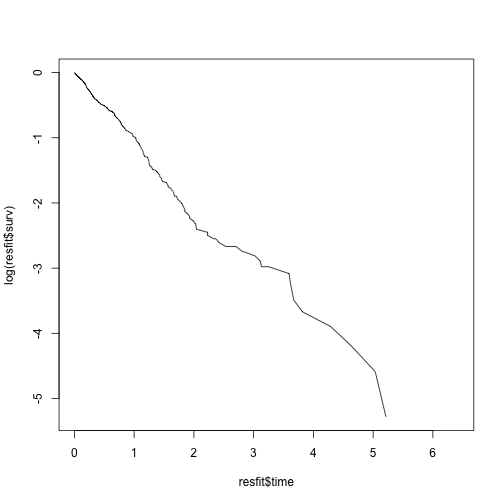# 6.2 Plot log-log surv against log time
plot(log(resfit$time), log(-log(resfit$surv)), type="l", xlab = "log time", ylab = "log-log Survival", main = "Halibut: Cox-Snell residuals")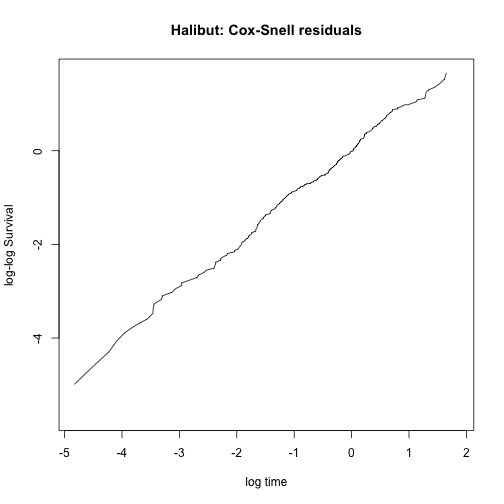## Martingale residuals

# Halibut data, continued

# Calculates martingale, deviance, score or Schoenfeld residuals for a Cox proportional hazards model.
martres = residuals(fitb, type="martingale")  # Martingale residuals
martres[1:3]

##          1          2          3
## -0.2384461 -0.1084664 -0.1073743

boxplot(martres ~ halibut$Towd, xlab="Towing duration", ylab="Martingale Residuals", boxwex=0.4) abline(h=0, lty=2)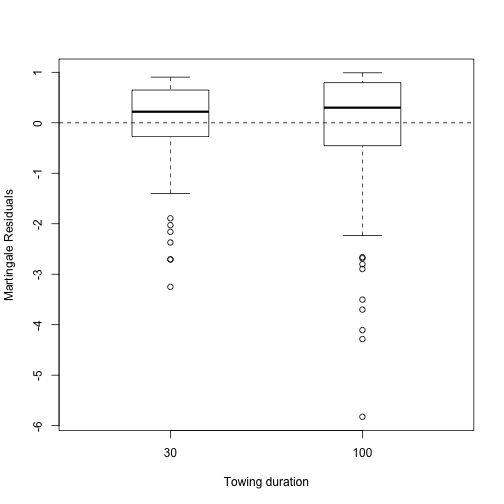plot(halibut$Length, martres, xlab="Halibut Length", ylab="Martingale Residuals", col=2)
abline(h=0, lty=2)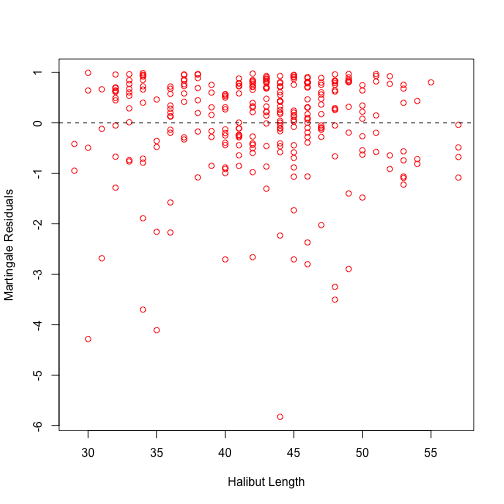plot(halibut$Handtime, martres, xlab="Handling Time", ylab="Martingale Residuals", col=3) abline(h=0, lty=2)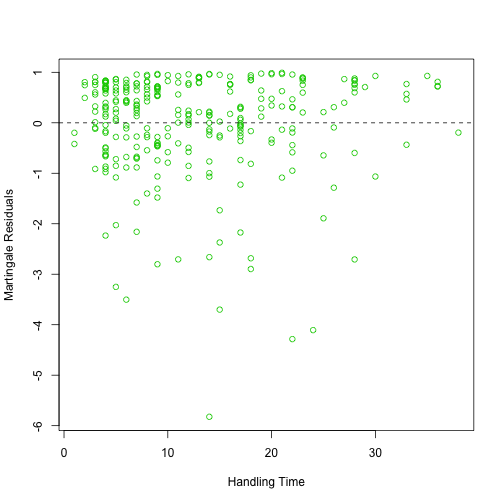plot(halibut$Logcat, martres, xlab="Log Catch Size", ylab="Martingale Residuals", col=4)
abline(h=0, lty=2)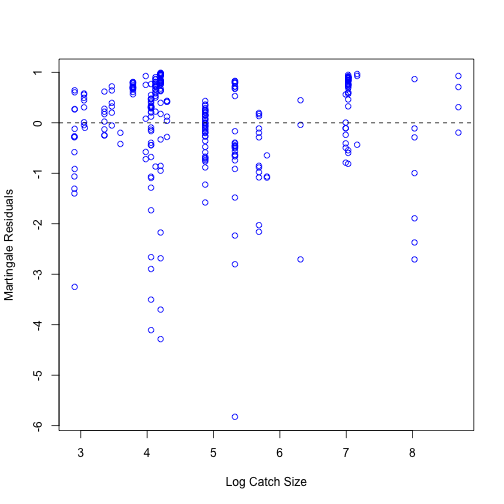plot(fitb$linear.predictors, martres, xlab="Linear Predictor", ylab="Martingale Residuals", col=6) abline(h=0, lty=2)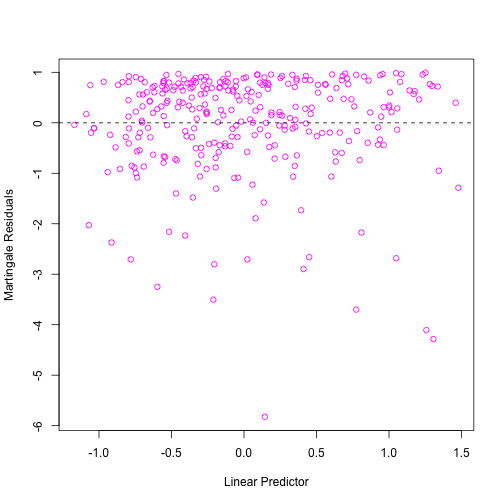## Deviance residuals # Halibut data, continued devres = residuals(fitb, type="deviance") # Deviance residuals devres[1:3]  ## 1 2 3 ## -0.2217595 -0.1047756 -0.1037553  boxplot(devres ~ halibut$Towd, xlab="Towing duration", ylab="Deviance Residuals", boxwex=0.4)
abline(h=0, lty=2)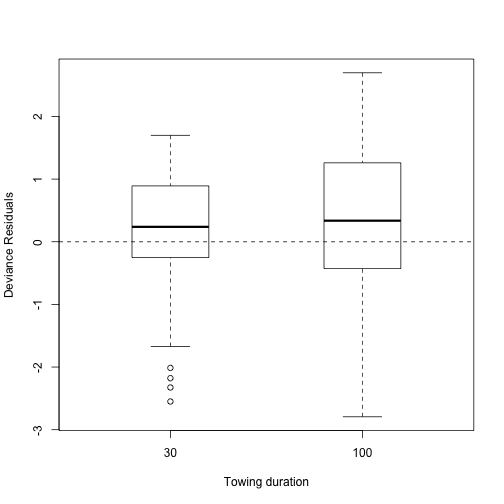plot(halibut$Length, devres, xlab="Halibut Length", ylab="Deviance Residuals", col=2) abline(h=0, lty=2)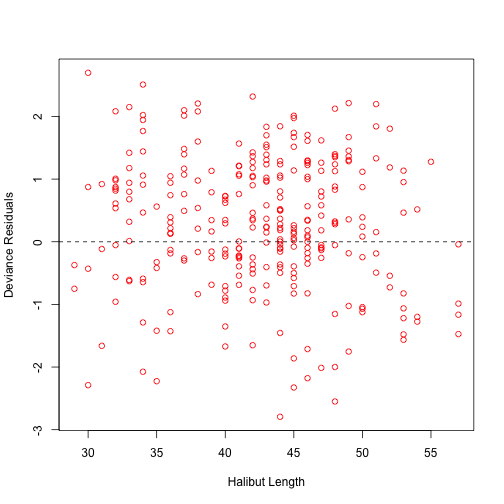plot(halibut$Handtime, devres, xlab="Handling Time", ylab="Deviance Residuals", col=3)
abline(h=0, lty=2)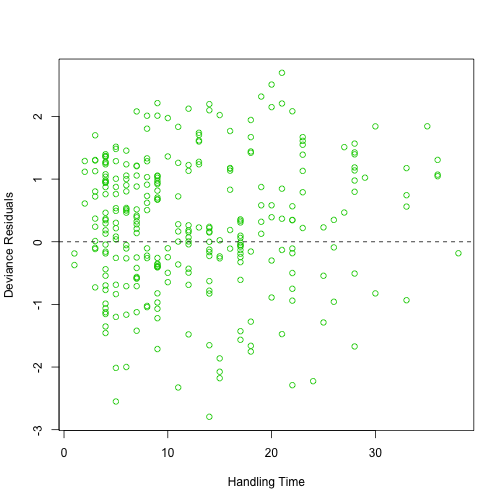plot(halibut$Logcat, devres, xlab="Log Catch Size", ylab="Deviance Residuals", col=4) abline(h=0, lty=2)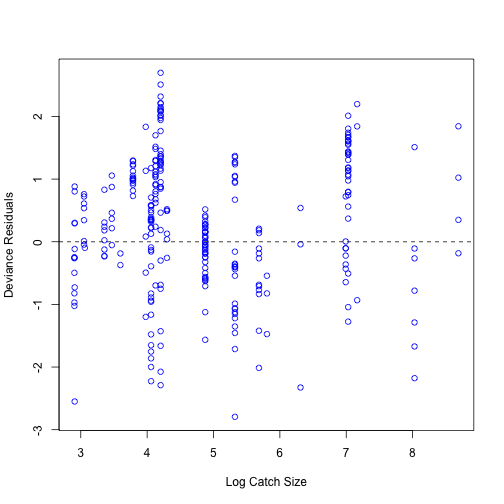plot(fitb$linear.predictors, devres, xlab="Linear Predictor", ylab="Deviance Residuals", col=6)
abline(h=0, lty=2)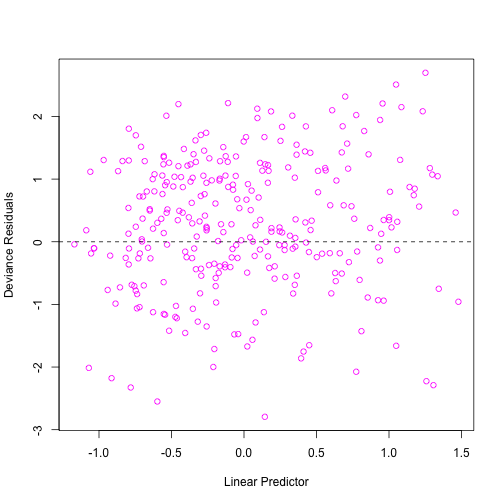## Schoenfeld residuals

# Halibut data, continued
schoeres = residuals(fitb, type="schoenfeld")  # Schoenfeld residuals
schoeres[1:3,]

##         Towd     Length Handtime     Logcat
## 1.1 13.59661 -10.584914 4.165367 -0.7233896
## 1.2 13.73103  -6.689555 3.206545 -0.7305410
## 1.3 14.00863   1.193347 2.279487 -0.7453105

evtime = sort(halibut$Time[halibut$Death==1]) # event times: 1.1,1.2,1.3 etc
plot(log(evtime), schoeres[,"Towd"], xlab="Log Survival time", ylab="Schoenfeld Residuals, Towing Duration")
abline(h=0, lty=2)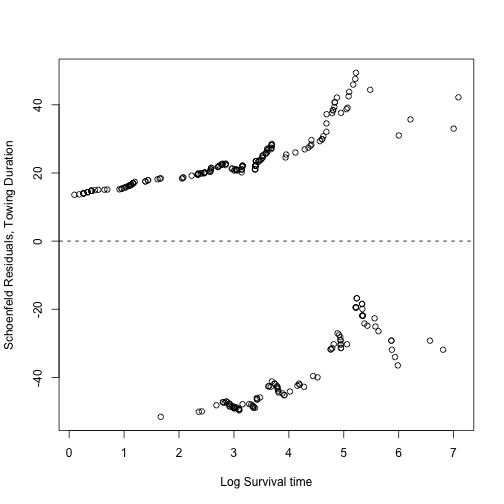plot(log(evtime), schoeres[,"Length"], xlab="Log Survival time", ylab="Schoenfeld Residuals, Fish Length", col=2)
abline(h=0, lty=2)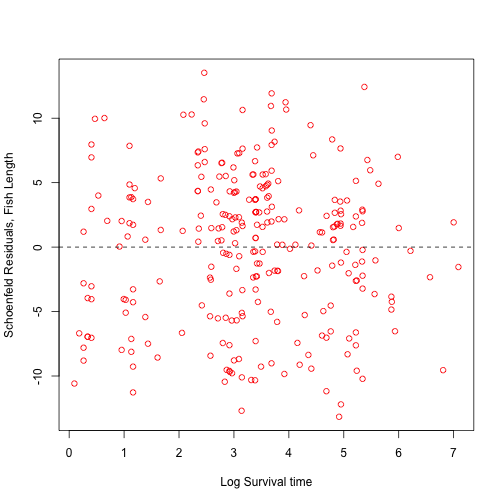plot(log(evtime), schoeres[,"Handtime"], xlab="Log Survival time", ylab="Schoenfeld Res, Handling Time", col=3)
abline(h=0, lty=2)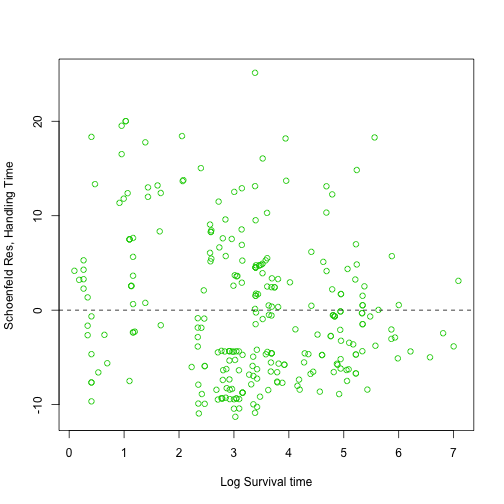plot(log(evtime), schoeres[,"Logcat"], xlab="Log Survival time", ylab="Schoenfeld Res, Log Catch Size", col=4)
abline(h=0, lty=2)